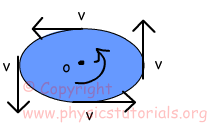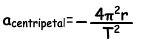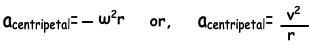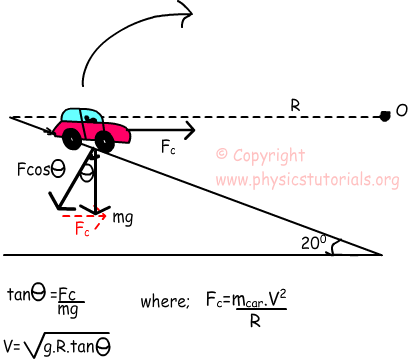Rotational Motion Cheatsheet

Rotational Motion Cheat Sheet

Tangential Speed (Linear Speed):

Linear speed and tangential speed gives the same meaning for circular motion. It can be defined as distance taken in a given time.If the object has one complete revolution then distance traveled becomes; 2πr which is the circumference of the circle object.

VA=2πr/time

Period: Time passing for one revolution is called period. The unit of period is second. T is the representation of period.

Frequency: Number of revolutions per one second. The unit of frequency is 1/second. We show frequency with letter f.

The equation of tangential speed becomes;

VA=2πr/T =2πrf

Angular Speed:

We define angular velocity as “change of the angular displacement in a unit of time”. One total rotation corresponds to 2π radians. Units of angular speed are revolution per unit time radians per second. We show angular speed with the Greek letter “ω” omega. All points on the platform have same angular velocity.

Average Speed=2π/T

ω=2π/T=2πf

Angular Acceleration:As you can see, direction of the speed changes as time passes and always tangent to the circle. Change in the direction of velocity means system has acceleration which is called angular acceleration. Because of the direction of acceleration, we call it centripetal acceleration.

Mathematical representation of centripetal acceleration is;“-“sign in front of the formula shows the direction with respect to the R position vector. We can rewrite centripetal acceleration in terms of angular velocity and tangential velocity.Centripetal Force: If there is acceleration then we can say there must be also a net force causing that acceleration. The direction of this net force is same as the direction of acceleration which is towards to the center. From the Newton’s Second Law of Motion;

F=m.a where; m is mass of the object, r is the radius of the circle, T is

Fc=-m4π²r/T² or the period, V is the tangential speed

Fc=mv²/r

Circular Motion on Inclined Planes

For having safe turn on the curve velocity of car must have the value given below which is the top limit.Centrifugal Force:

When a car goes around a curve we feel that as if something pulls us outward to the center of that curve. In real, of course there is no such a force exerting on us. Because of the Newton’s First Law of Motion “Law of Inertia”, we feel that something pulls us outward. This is only inertia of us, in real there is no centrifugal force.

Torque

We define torque as the capability of rotating objects around a fixed axis. In other words, it is the multiplication of force and the shortest distance between application point of force and the fixed axis. From the definition, you can also infer that, torque is a vector quantity both having direction and magnitude. . Torque is shown in physics with the symbol “τ”.You can come across torque with other name “moment”. We calculate torque with following formula;

τ=Applied Force.Distance.sinӨ

Rotational Motion Exams and Solutions

Author: